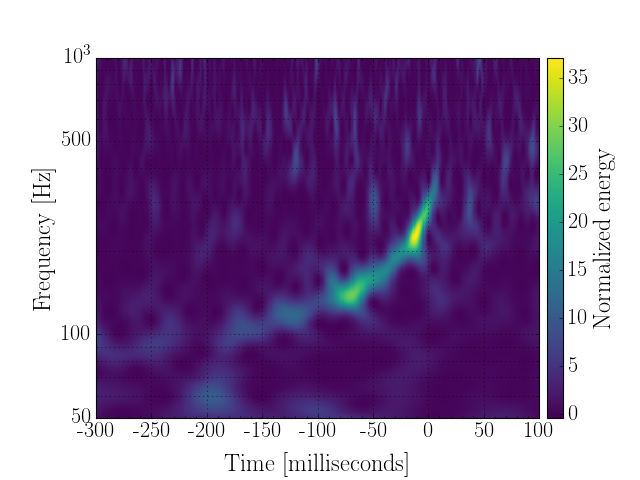# Generate the Q-transform of a `TimeSeries`¶

First, we identify the GPS time of interest:

```gps = 968654558
```

and use that to define the start and end times of our required data

```duration = 32
start = int(round(gps - duration/2.))
end = start + duration
```

next, we import the `TimeSeries` and fetch some open data from LOSC:

```from gwpy.timeseries import TimeSeries
data = TimeSeries.fetch_open_data('H1', start, end)
```

and next we generate the `q_transform` of these data:

```qspecgram = data.q_transform()
```

Now, we can plot the resulting `Spectrogram`, focusing on a specific window around the interesting time

Note

Using `crop` is highly recommended at this stage because rendering the high-resolution spectrogram as it is done here is very slow (for experts this is because we’re using `pcolormesh` and not any sort of image interpolation, mainly to support both linear and log scaling nicely)

```plot = qspecgram.crop(gps-.3, gps+.1).plot(figsize=[8, 6])
ax = plot.gca()
ax.set_epoch(gps)
ax.set_yscale('log')
ax.set_xlabel('Time [milliseconds]')
ax.set_ylim(50, 1000)
ax.grid(True, axis='y', which='both')